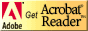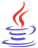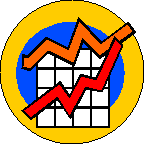# Standardized Test Preparation and Tips for Success

## Strand Resources: Data Analysis and ProbabilityCT4ME has identified resources related to each benchmark of the Ohio Mathematics Academic Content Standards that students should have mastered by the end of the grades 8-10 program.

Identify the benchmark (A-K) for review in Data Analysis and Probability. Below each benchmark, you will find Web resources for reviewing the concept and practice problems.

Students should be able to review on their own using these resources.  If you are not an Ohio educator, these resources can still benefit your test prep efforts, as benchmarks in other states are similarly stated.

Data Analysis and Probability (pdf)  This test prep booklet (about 200 KB) is designed to help you monitor your progress toward mastery of each benchmark in this strand.  You can print the entire booklet, or just those pages for benchmarks you need to work on.  Write notes in the booklet next to each resource you use in your review.If needed, download Adobe Acrobat Reader, free software for viewing and printing PDF files.You might need to download Java, a free plug-in to interact with virtual manipulatives on this page.Other strand resources:

Number, Number Sense, and Operations ]   [ Measurement ]   [ Geometry and Spatial Sense ]   [ Patterns, Functions, and Algebra ]   [ Data Analysis and Probability ]   [ Mathematical Processes ]### What do we mean by Data and Probability?### Benchmarks in Data Analysis and Probability

Benchmarks in this strand have been correlated to the Common Core State Standards (CCSS) for Mathematics for the Conceptual Category (CC), Domain (D), and Cluster (C).A.  Create, interpret and use graphical displays and statistical measures to describe data; e.g., box-and-whisker plots, histograms, scatterplots, measures of center and variability.

CCSS: CC: Statistics and Probability, D: Interpreting Categorical and Quantitative Data, B: Summarize, represent, and interpret data on two categorical and quantitative variables.

CCSS: CC: grade 7, D: Statistics and Probability, C: Draw informal comparative inferences about two populations.

CCSS: CC: grade 8, D: Statistics and Probability, C: Investigate patterns of association in bivariate data.

Line graphs and histograms are only used with continuous data, which means that theoretically all values are possible (no gaps) in an interval (between any two it is possible to get another).  Examples include height, weight, time to complete your homework, and time to complete a trip.  Line graphs are good to demonstrate trends, such as sales in a company over time or changes in temperature over time.Bar graphs and circle graphs (often called pie charts) are only used with discrete data, which means that only certain values (gaps) are possible. Examples typically include counting, as in the number of students in a class, the number of crimes reported to the police, or the number of tickets sold to a game.  Note that pie charts are good for presenting percentages.  Bar graphs can be presented vertically or horizontally.  For example, a horizontal bar graph could be used to compare expenses among four departments of a company.Scatter plots are used for experimental data to determine if there is a relationship between the variables studied.When creating graphs, note the following 10 common errors, provided by CanTeach (CA):

1. No title on graph
2. Source of data not given
3. Pictograph - no key
4. Scales are interrupted
5. Scales are not labeled
6. Symbols in pictographs not same size or equally spaced on graph
7. Use of 2 or 3 dimensional objects to compare data (area/volume)
8. Scales do not start at zero
9. Numbers on axis (vertical or horizontal) not equally spaced
10. Scale is selected to produce desired result

The basics about line graphs in representing data is a short module by David Lane.  It shows how line graphs compare to bar graphs and when to use line graphs.  Two self-check questions included.

National Center for Education Statistics (for students): Create a Graph

Statistics Canada: Statistics, Power from Data: Graph Types and create your graph.

Utah State University, National Library of Virtual Manipulatives:Play the YouTube videos from the Ohio Resource Center Tutorials for High School Mathematics:

• Data Display for organizing information into tables and graphs with titles, legends, correct units, error bars, and fitting functions.

• Lines of Fit for defining and finding lines of best fit using real data.

B.  Evaluate different graphical representations of the same data to determine which is the most appropriate representation for an identified purpose.

CCSS: CC: Statistics and Probability, D: Interpreting Categorical and Quantitative Data, C: Summarize, represent, and interpret data on a single count or measurement variable.Play the YouTube video from the Ohio Resource Center Tutorials for High School Mathematics:

• Graphing Data for working with a spreadsheet to create different types of graphs with discussion of appropriateness.

C.  Compare the characteristics of the mean, median and mode for a given set of data, and explain which measure of center best represents the data.

CCSS: CC: grade 6, D: Statistics and Probability, C: Summarize and describe distributions.

CCSS: CC: grade 7, D: Statistics and Probability, C: Draw informal comparative inferences about two populations.

Statistics Canada: Statistics, Power from Data: Measures of Central Tendency

University of Illinois at Urbana-Champaign, Jay Hill: Introduction to Descriptive Statistics--mean, median, mode, range, and so on, simply explained.

BBC GCSE Bitesize Maths, Statistics and Probability: Averages: Mean, Mode, and Median --There is an explanation (called Revise), activity, and test.

D.  Find, use and interpret measures of center and spread, such as mean and quartiles, and use those measures to compare and draw conclusions about sets of data.

CCSS: CC: Statistics and Probability, D: Interpreting Categorical and Quantitative Data, C: Summarize, represent, and interpret data on a single count or measurement variable.

Shodor Interactivate: Introduction to Statistics: Mean, Median, Mode

AlgebraLab.org: Mean, Median, Mode.  Lesson, interactive online practice problems.  Show the Related AlgebraLab documents for activities, additional practice problems and word problems.

Play the YouTube video from the Ohio Resource Center Tutorials for High School Mathematics:Play video at YouTube.com:

• GCSE Maths Median and IQR: This short video shows how to find a median, lower quartile, upper quartile, then inter-quartile range.

E.  Evaluate the validity of claims and predictions that are based on data by examining the appropriateness of the data collection and analysis.

CCSS: CC: Statistics and Probability, D: Making Inferences and Justifying Conclusions, C: Make inferences and justify conclusions from sample surveys, experiments, and observational studies.

Statistics How To: Misleading Graphs: Real Life Examples

BBC (GCSE Bitesize): Statistics and Probability: Collecting Data, Averages, Representing Data

F.  Construct convincing arguments based on analysis of data and interpretation of graphs.

CCSS: CC: Statistics and Probability, D: Making Inferences and Justifying Conclusions, B: Make inferences and justify conclusions from sample surveys, experiments, and observational studies.

Interpret line graphs from the Earth Observatory at NASA.  Analyze some temperature and precipitation graphs from different cities and match them up to the correct biome.

Describe sampling methods and analyze the effects of method chosen on how well the resulting sample represents the population.

CCSS: CC: Statistics and Probability, D: Making Inferences and Justifying Conclusions, A: Understand and evaluate random processes underlying statistical experiments.

CCSS: CC: grade 7, D: Statistics and Probability, C: Use random sampling to draw inferences about a population.

Statistics Canada: Statistics: Power from Data: Sampling Methods

H.  Use counting techniques, such as permutations and combinations, to determine the total number of options and possible outcomes.

CCSS: CC: Statistics and Probability, D: Conditional Probability and Rules of Probability, C: Use the rules of probability to compute probabilities of compound events in a uniform probability model.

Webmath.com: Permutations Verify your list of permutations for up to 8 entries.

I.  Design an experiment to test a theoretical probability, and record and explain results.

CCSS: CC: Statistics and Probability, D: Use Probability to Make Decisions, B: Use probability to evaluate outcomes of decisions.

AlgebraLab.org: Law of Large Numbers and Simulations explains the process of experimental probability and suggests experiments to try.  Be sure to show related AlgebraLab documents for additional lessons and practice problems in probability.

J.  Compute probabilities of compound events, independent events, and simple dependent events.

CCSS: CC: Statistics and Probability, D: Conditional Probability and Rules of Probability, C: Understand independence and conditional probability and use them to interpret data, and C: Use the rules of probability to compute probabilities of compound events in a uniform probability model.

CCSS: CC: grade 7, D: Statistics and Probability, C: Investigate chance processes and develop, use, and evaluate probability models.

Integrated Publishing: Dependent Events, explanation of probability and problemsPlay videos at YouTube.com:

• GCSE Probability Part 1: This video is from a professor in Great Britain who nicely explains probability and shows examples: expected frequency, the typical problems involving picking balls from a bag, mutually exclusive events, independent events, and drawing diagrams to help identify possibilities.

• Probability Part 2: tree diagrams.  The same professor in Great Britain discusses the balls in a bag problems when making two picks, which leads to tree diagrams to help determine probability involving replacing after each pick, and then not replacing after a pick.

K.  Make predictions based on theoretical probabilities and experimental results.

CCSS: CC: Statistics and Probability, D: Use Probability to Make Decisions, A: Calculate expected values and use them to solve problems.

Utah State University, National Library of Virtual Manipulatives: Box Model -- investigate theoretical and experimental probabilities.Are you ready for the test?

#### References

*Ohio Mathematics Academic Content Standards (2001). http://www.ode.state.oh.us/GD/Templates/Pages/ODE/ODEPrimary.aspx?page=2&TopicID=1696&TopicRelationID=1704 [URL is no longer active.]

Ohio Mathematics Academic Content Standards Crosswalk, K-8 and High School (2010). https://web.archive.org/web/20180206120934/http://education.ohio.gov/getattachment/Topics/Ohio-s-New-Learning-Standards/Mathematics/Transition-Tools-Ohio-s-New-Learning-Standards-K-1/Alignment-Toolkit-Phase-1-Gap-Analysis/HS-crosswalk-mathematics-4-17-12.pdf.aspx [Revised 4/17/2012].

*Note 2019: The current academic content standards for mathematics are now available at http://education.ohio.gov/Topics/Learning-in-Ohio/Mathematics/Ohio-s-Learning-Standards-in-MathematicsSee other strand resources:

Number, Number Sense, and Operations ]   [ Measurement ]   [ Geometry and Spatial Sense ]   [ Patterns, Functions, and Algebra ]   [ Data Analysis and Probability ]   [ Mathematical Processes ]See related pages: [ Standardized Test Preparation and Tips for Success (first  page)]  [ OGT Introduction ]